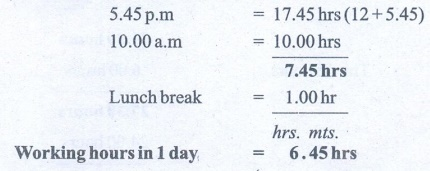Home | | Maths 6th Std | Exercise 2.3

# Exercise 2.3

6th Maths : Term 2 Unit 2 : Measurements : Exercise 2.3 : Miscellaneous practice Problems, Challenge Problems, Text Book Back Exercises Questions with Answers, Solution

Exercise 2.3

Miscellaneous practice Problems

1. Two pipes whose lengths are 7 m 25 cm and 8 m 13 cm joined by welding and then a small piece 60 cm is cut from the whole. What is the remaining length of the pipe?Length ofthe remaining pipe = 14 m 78 cm

2. The saplings are planted at a distance of 2 m 50 cm in the road of length 5 km by saravanan. If he has 2560 saplings, how many saplings will be planted by him? how many saplings are left?Length of the road = 5 km = 500000 cm

Distance between each sapling = 2 m 50 cm = 250 cm

Number of saplings planted = 500000 ÷ 250 = 2000

Number of saplings Saravanan has = 2560

Number of saplings planted = 2000

Number of saplings left = 560

3. Put mark in the circles which adds upto the given measure.4. Make a calendar for the month of February 2020. (Hint: January 1st 2020 is Wednesday)

January First Wednesday

31st Friday

2020 is leap year.

February 20205. Observe and Collect the data for a minute:i. Number of times a person breathes 45

ii. Number of times heart beats 72

iii. Number of times the eyes blink 54

iv. Distance by walking 50 m

v. Distance by running 200m

vi. Number of situps 25

vii. Number of claps 137

viii. Number of lines to write 4

ix. Number of lines to read 7

x. Number of Tamil verbs to say 23Challenge Problems

6. A squirrel wants to eat the grains quickly. Help the Squirrel to find the shortest way to reach the grains. ( Use your scale to measure length of the line segments)AB + BC + CD + DE

(1.7 + 2.2 + 2 + 1.7) cm = 7.6 cm

AG + GF + FE

(2.2 + 1.5 + 1.5 + 2.3) cm = 7.5 cm

AH + HI + IJ + JE

(2.5 + 2 + 0.7 + 2.7) cm = 7.9 cm

The shortest way to reach the grains is the way from A through G, F and reach E.

7. A room has a door whose measures are 1 m wide and 2 m 50 cm high.

i. Can we take a bed of 2 m and 20 cm length and 90 cm wide into the room.?

Yes. We can take the bed into the room. Because the length and wide of the bed is smaller than the wide and height of the door.

8. A post office functions from 10 a.m to 5.45 p.m with a lunch break of 1 hour. If the post office works for 6 days a week, find the total duration of working hours in a week.

The duration of working hours in one dayWorking hours in 1 day = 6.45 hrsWorking hours in 6 days = 6.45 × 6

= 36 hrs 270 mts.

= 36 hrs + 4 hrs 30 minutes

= 40 hrs 30 minutes

Total duration of working hours in a week = 40 hours 30 minutes.

9. Seetha wakes up at 5.20 a.m. She spends 35 minutes to get ready and travels 15 minutes to reach the railway station. If the train departs exactly at 6:00 a.m, will Seetha catch the train?

Seetha wakes up at 5.20 am = 5 hours 20 minutes

Time taken to get ready = 35 minutes

Traveling Time = 15 minutes

The time she reached the railway station = 5 hours 70 minutes

= 5hrs + (60 +10) minutes

= 6 hours 10 minutes

The train departure time = 6 hours

So, Seetha will not catch the train

10. A doctor advised Vairavan to take one tablet every 6 hours once in the 1st day and once every 8 hours on the 2nd and 3rd day. If he starts to take 9.30 a.m first dose. Prepare a time chart to take tablet in railway time.

First day Vairavan took tablet at 9.30 a.m. = 9.30 hoursTags : Questions with Answers, Solution | Measurements | Term 2 Chapter 2 | 6th Maths , 6th Maths : Term 2 Unit 2 : Measurements
Study Material, Lecturing Notes, Assignment, Reference, Wiki description explanation, brief detail
6th Maths : Term 2 Unit 2 : Measurements : Exercise 2.3 | Questions with Answers, Solution | Measurements | Term 2 Chapter 2 | 6th Maths